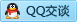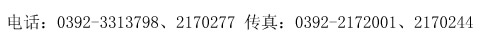# 煤质化验数据计算器

 水分分析（依据GB/T212空气干燥法） 挥发分分析（依据GB/T212标准） 称量瓶重m0= g 坩埚重m0= g 煤样重m= g 煤样重m= g 称量瓶加煤样重m3= g 实验后坩埚加煤样重m3= g 水分Mad % 挥发分Vad % 灰分分析（依据GB/T212标准） 氮分析（依据GB476凯氏蒸馏法） 灰皿重m0= g 煤样重m= g 煤样重m= g 硫酸标准溶液的浓度C= mol/L 实验后灰皿加煤样重m3= g 硫酸标准溶液的用量V1= mL 灰分Aad % 空白实验时硫酸标准溶液的用量V2= mL 固定碳Fcad % 氮Nad % 碳酸盐二氧化碳（CO2）ad（依据GB/T218标准） 结渣性分析Clin(采用GB/T1572标准) 试验前U形管的总质量m1= g 粒度大于6mm渣块的质量G1= g 试验后U形管的总质量m2= g 总灰渣质量G= g 空白值m3= g 结渣率Clin % 空气干燥煤样的质量m= g 氧分析Oad（依据GB476标准） 碳酸盐二氧化碳含量[CO2]ad % 全硫含量Sad = % 氧含量Oad % 二氧化碳反应性(采用GB/T2006标准) 机械强度(采用GB/T2006标准) 钢瓶二氧化碳气体中杂质气体含量a= % 落下实验后大于25mm块煤质量m1= kg 反应后气体中二氧化碳含量V= % 落下实验前块煤质量m= kg 二氧化碳还原率 % 煤的机械强度SS % 碳氢分析（依据GB476燃烧吸收法） 罗加指数分析R.I.(采用GB5449标准) 吸收二氧化碳的U形管的增重m1= g 第一次转鼓试验前筛上焦炭重量m11= g 吸收水分的U形管的增重m2= g 第一次转鼓试验后筛上焦炭重量m1= g 水分空白值 m3= g 第二次转鼓试验后筛上焦炭重量m2= g 煤样的质量m= g 第三次转鼓试验后筛上焦炭重量m3= g 碳含量Cad % 焦化后焦炭的总重量m= g 氢含量Had % 罗加指数R.I. % 粘结指数 G (采用GB5447标准) 粘结指数 G<18 (采用GB5447标准) 第一次转鼓试验后.筛上部分重量m1= g 第一次转鼓试验后.筛上部分重量m1= g 第二次转鼓试验后,筛上部分重量m2= g 第二次转鼓试验后,筛上部分重量m2= g 焦化处理后焦渣总重m= g 焦化处理后焦渣总重m= g 粘结指数 G % 粘结指数 G<18 % 低温干馏实验(采用GB480标准) 浮沉实验(采用GB478标准) 空气干燥煤样的质量m= g 浮物质量A 冷凝物质量m1= g 中间物质B 干馏总水分质量m2= g 沿下物质C 半角总质量m3= g 浮物质量 % 焦油产量 Tarad % 中间物质 % 干馏总水分产率 Water ad % 沉物质量 % 热解水产率Wp.ad % 半角产率Cokead % 煤的热稳定性测定实验（采用GB1573标准） 煤的哈磨指数实验（采用GB2565标准） 残焦质量G= g 煤样质量m= g 各级残焦质量之和= g 筛上物质量m1= g 煤的热稳定性TS % 筛下物质量m2 g 烟煤发热量 无烟煤发热量 灰分Aad= % 灰分Aad= % 全水分Mar= % 全水分Mar= % 分析水Mad= % 分析水Mad= % 挥发分Vad= % 挥发分Vad= % 焦渣特性= 分析基低位发热量 cal/g 分析基低位发热量 cal/g 收到基低位发热量 cal/g 收到基低位发热量 cal/g